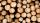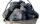Milimeters

The pool is 6 meters long, 3 meters wide and the water in it is filled with water to a height 1.9 m. When John jumped into it and completely submerged, the level has risen by 4.2 mm. How much weight John when we know that one liter of the human body weighs approximately one kilogram?

Result

m =  75.6 kg

Solution:Leave us a comment of example and its solution (i.e. if it is still somewhat unclear...):Be the first to comment!To solve this example are needed these knowledge from mathematics:

Do you know the volume and unit volume, and want to convert volume units?

Next similar examples:

1. Water poolWhat water level is in the pool shaped cuboid with bottom dimensions of 25 m and 10 meters, when is 3750hl water in the pool.
2. Pool in litresPool has a width of 3.5 m length of 6 m and a height 1.60 meters. Calculate pool volume in liters.
3. SnowSnow fell overnight layer of thickness 19 cm. In the morning I had to clear a path 69 m long and one meter wide. How many cubic meters of snow I clear? How many kilos was it? (1 m3 fresh snow weighs 350 kg)
4. Glass doorWhat is the weight of glass door panel 5 mm thick height 2.1 meters and a width of 65 cm and 1 cubic dm of glass weighs 2.5 kg?
5. Fire tankHow deep is the fire tank with the dimensions of the bottom 7m and 12m, when filled with 420 m3 of water?
6. TankTo cuboid tank whose bottom has dimensions of 9 m and 15 m were flow 1080 hectoliters of water. This was filled 40% of the tank volume. Calculate the depth of the tank.
7. AquariumAquarium is rectangular box with square base containing 76 liters of water. Length of base edge is 42 cm. To what height the water level goes?
8. Water reservoirThe water tank has a cuboid with edges a= 1 m, b=2 m , c = 1 m. Calculate how many centimeters of water level falls, if we fill fifteen 12 liters cans.
9. Cylindrical tank 2If a cylindrical tank with volume is used 12320cm raised to the power of 3 and base 28cm is used to store water. How many liters of water can it hold?
10. HectolitersHow many hectoliters of water fits into cuboid tank with dimensions of a = 3.5 m b = 2.5 m c = 1.4 m?
11. The shopThe shop has 3 hectoliters of water. How many liter bottles is it?
12. Concrete boxThe concrete box with walls thick 5 cm has the following external dimensions: length 1.4 m, width 38 cm and height 42 cm. How many liters of soil can fit if I fill it to the brim?
13. Oak cuboidOak timber is rectangular shaped with dimensions of 2m, 30 cm and 15 cm. It weight is 70 kg. Calculate the weight 1 dm³ of timber.Added together and write as decimal number: LXVII + MLXIVWood density is 0.6 g/cm3. How many kilograms weight 1 m3 of wood?Find the volume of cuboidal box whose one edge is: a) 1.4m and b) 2.1dmCoal hopper has a capacity of 285 liters. How many tons is it? The bulk density of coal is 916 kg/m3.